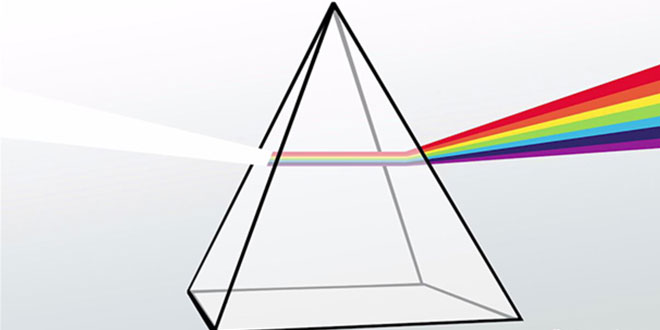Tuesday , July 5 2022# NCERT 8th Class (CBSE) Science: Light

#### Question: What is reflection of light?

Answer: The objects send back the light which falls on them. This sending back of light by objects is called reflection of light.

#### Question:What is the second law of reflection?

Answer: The second law of reflection states that the incident ray, the normal and the reflected ray all lie in the same
plane.

#### Question: What is lateral inversion?

Answer: The phenomenon of reversal of right and left sides in the mirror image formed by a plane mirror, is called lateral inversion. The lateral inversion in the mirror image is due to the reflection of light.

#### Question: Draw the image of the letter ‘P’ as formed by a plane mirror placed on the right side of ‘P’.#### Question: What is the function of a periscope in a submarine?

Answer: From a submarine under the sea, a sailor can see objects and enemy ships on the surface of the sea by using periscope.

#### Question: Differentiate between regular and diffused reflection. Draw diagrams to show the two types of reflections.

Regular reflection
Diffused reflection
1. If a beam of light falls on it, the entire beam is reflected in one direction.
1. If a beam falls on a uneven surface they get reflected in different direction.
2. It is reflected on a smooth surface.
2. It is reflected on an uneven surface.
3. Diagram
3. Diagram

#### Question: Why are multiple images formed when two mirrors are placed at right angles to each other?

Answer: When two mirrors are placed at angles to each other we get multiple images because the image formed by one mirror acts as an object for the second mirror. Further images of an image are also formed. This continues till no more reflection by either mirror is possible. Two mirrors placed at right angles (90°) to each other will form actually 3 images.

#### Question: Calculate the number of images formed by plane mirrors kept at an angle of 10° to each other.

Answer: The number of images formed by mirrors at an angle is n = (360/0) — 1 where 0 = angle between the mirrors. Here, 0 = 10°. So, n = (360/10) — 1 = 36 — 1 = 35. Hence, the number of images will be 35.

## Sources of Energy: 10th Science Chapter 14

Class: 10th Class Subject: Science Chapter: Chapter 14: Sources of Energy Quiz: – Questions MCQs: …